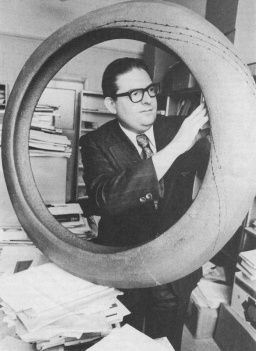Enter the content which will be displayed in sticky bar

# MemberDr. Robert W. Bass
local time: 2021-11-28 05:51 (-07:00 DST)
Dr. Robert W. Bass (Abstracts)
Titles
Abstracts Details

The following protocol is designed to be intuitively convincing to a layman who, if told that needles 2, 3 and 4 have been pulsed with constant-voltage DC-electricity for twice, thrice and quadruple the amount of time as needle 1, and then measured to contain twice, thrice and quadruple the amount of He4 as the first needle, will instantly reject any doubt that the electrical pulse was creating He4 from some form of nuclear-chemistry process whose action was directly proportional to the amount of DC electrical energy used. At the same time, the protocol is sufficiently statistically sophisticated in its rigorous application of Experiment Design theory as to satisfy the most skeptical and informed critic.

In a Newtonian model of e.g. the Bohr atom, it is physically incorrect to omit the aggregate forces from all electrostatic charges (monopole radiation [with or without retarded potentials]) in the visible universe. Though the standard hypotheses or cosmic homogeneity/isotropy imply such forces have a zero-mean, they also have a non-zero variance! It is known since Fenyes (1952) & Nelson (1967, 1985) that Schrodinger's Equation is derivable from Newtonian mechanics, in Stochastic Mechanics (SM) as a neo-classical alternative to the Copenhagen interpretation of QM. In SM, the forces acting are augmented by a zero-mean, non-zero-variance background-field process, but (although Nelson remarks that "no physical system ... is truly isolated") no plausible candidate for the source of mutual energy transfer which averages out to zero" and is thus "responsible for ... quantum fluctuations" has hitherto been identified.  Here it is proved that the cosmoc monopole radiations from all of the universe's quarks & electroncs provide a quantitatively adequate source of Schrodinger's micro-physical zitterbewegungen, thereby explaining both the necessity of a statistical treatment of microphysical "jittering" and rendering mistaken/irrelevant the oft-noted "weirdness" of reality alleged by Copenhagen. For non-relativistic motions, [] in SM, where M, x, and F have classical Newtonian significance, and where if the mass M carries one electron charge, the force-vector F is the sum of the electrostatic forces of all quarks & electrons in a sphere of radius Ro = 1 Astronomical Unit (AU), while w(t) is a unit-intensity-matrix stochastic vector-process (having dimensions 1/{m2 - sec] and h is a scale-factor having units of angular momentum. Specifically, if E is the expectation operator and [] the Dirac delta-function, E{W(t)} = 0 and E [], where I3 is an identity 3-matrix with units 1/[m2 - sec]. Summing the aggregate cosmic forces from outside said sphere arising from charge-sources of mean number density  [] particles/m3 it is easy to compute (from a convergent integral) that

[]

where e denotes the electron charge and [] the permittivity of free space and [] a unit-intensity scale-factor of dimensions (m5/2.sec). Now [] per m3 from standard physical cosmology, where [] denotes the usual critical density ratio, and where Ho denotes the value, at the present epoch, in (km/sec)/Mpc, of Hubble's constant. To test validity of the preceding derivation, note that identifying h with Plank's constant yields [] = 0.0693, in relatively excellent agreement with the latest observational data. The result renders the Copenhagen interpretation untenable, vindicates Einstein's surmise that "Goe does not play dice," and demonstrates the ontological reality (& physical origin) of non-virtual Zero-Point Fluctuations (ZPF).